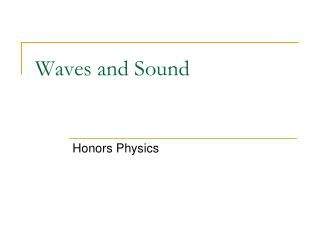Download PresentationWaves and Sound

# Waves and Sound

Download Presentation## Waves and Sound

- - - - - - - - - - - - - - - - - - - - - - - - - - - E N D - - - - - - - - - - - - - - - - - - - - - - - - - - -
##### Presentation Transcript

1. Waves and Sound Honors Physics

2. What is a wave A WAVE is a vibration or disturbance in space. A MEDIUM is the substance that all SOUND WAVES travel through and need to have in order to move.

3. Two types of Waves The first type of wave is called Longitudinal. Longitudinal Wave - A fixed point will move parallel with the wave motion 2 areas Compression- an area of high molecular density and pressure Rarefaction - an area of low molecular density and pressure

4. Two types of Waves The second type of wave is called Transverse. Transverse Wave - A fixed point will move perpendicular with the wave motion. Wave parts(recall demo for simple harmonic motion )- crest, trough, wavelength, amplitude, frequency, period

5. Wave Speed You can find the speed of a wave by multiplying the wave’s wavelength in meters by the frequency (cycles per second). Since a “cycle” is not a standard unit this gives you meters/second.

6. Example A harmonic wave is traveling along a rope. It is observed that the oscillator that generates the wave completes 40.0 vibrations in 30.0 s. Also, a given maximum travels 425 cm along a rope in 10.0 s . What is the wavelength? 1.33 Hz 0.425 m/s 0.319 m

7. Standing Waves A standing wave is produced when a wave that is traveling is reflected back upon itself. There are two main parts to a standing wave: • Antinodes – Areas of MAXIMUM AMPLITUDE • Nodes – Areas of ZERO AMPLITUDE.

8. Sound Waves Sound Waves are a common type of standing wave as they are caused by RESONANCE. Resonance – when a FORCED vibration matches an object’s natural frequency thus producing vibration, sound, or even damage. One example of this involves shattering a wine glass by hitting a musical note that is on the same frequency as the natural frequency of the glass. (Natural frequency depends on the size, shape, and composition of the object in question.) Because the frequencies resonate, or are in sync with one another, maximum energy transfer is possible.

9. Sound Waves The production of sound involves setting up a wave in air. To set up a CONTINUOUS sound you will need to set a standing wave pattern. Three LARGE CLASSES of instruments • Stringed - standing wave is set up in a tightly stretched string • Percussion - standing wave is produced by the vibration of solid objects • Wind - standing wave is set up in a column of air that is either OPEN or CLOSED Factors that influence the speed of sound are density of solids or liquid, and TEMPERATURE

10. Closed Pipes Have an antinode at one end and a node at the other. Each sound you hear will occur when an antinode appears at the top of the pipe. What is the SMALLEST length of pipe you can have to hear a sound? You get your first sound or encounter your first antinode when the length of the actual pipe is equal to a quarter of a wavelength. This FIRST SOUND is called the FUNDAMENTAL FREQUENCY or the FIRST HARMONIC.

11. Closed Pipes - Harmonics Harmonics are MULTIPLES of the fundamental frequency. In a closed pipe, you have a NODE at the 2nd harmonic position, therefore NO SOUND is produced

12. Closed Pipes - Harmonics In a closed pipe you have an ANTINODE at the 3rd harmonic position, therefore SOUND is produced. CONCLUSION: Sounds in CLOSED pipes are produced ONLY at ODD HARMONICS!

13. Open Pipes OPEN PIPES- have an antinode on BOTH ends of the tube. What is the SMALLEST length of pipe you can have to hear a sound? You will get your FIRST sound when the length of the pipe equals one-half of a wavelength.

14. Open Pipes - Harmonics Since harmonics are MULTIPLES of the fundamental, the second harmonic of an “open pipe” will be ONE WAVELENGTH. The picture above is the SECOND harmonic or the FIRST OVERTONE.

15. Open pipes - Harmonics Another half of a wavelength would ALSO produce an antinode on BOTH ends. In fact, no matter how many halves you add you will always have an antinode on the ends The picture above is the THIRD harmonic or the SECOND OVERTONE. CONCLUSION: Sounds in OPEN pipes are produced at ALL HARMONICS!

16. Example The speed of sound waves in air is found to be 340 m/s. Determine the fundamental frequency (1st harmonic) of an open-end air column which has a length of 67.5 cm. 251.85 HZ

17. Example The windpipe of a typical whooping crane is about 1.525-m long. What is the lowest resonant frequency of this pipe assuming it is a pipe closed at one end? Assume a temperature of 37°C. 353.2 m/s 57.90 Hz